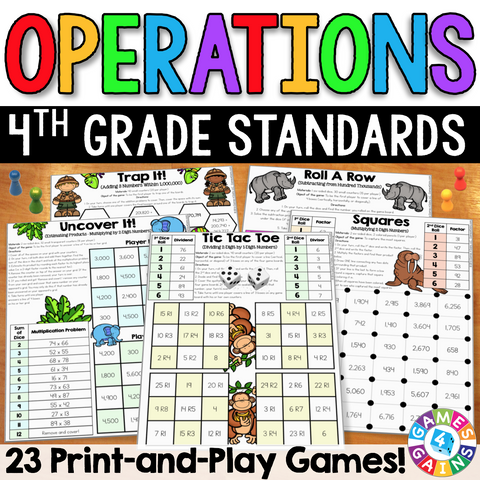• \$695

These games make 4th grade operations standards so much fun to practice! This 4th Grade Operations Games Pack contains 23 fun and engaging printable board games to help students to practice 4th grade addition, subtraction, multiplication, and division standards.

This pack includes 23 DIFFERENTIATED games that cover the following 4th grade operations skills:

• Estimating Sums Within 1,000,000
• Adding 2 Numbers Within 1,000,000
• Adding 3 Numbers Within 1,000,000
• Determining the Unknown in Addition Problems Within 1,000,000

Subtraction:
• Estimating Differences Within 1,000,000
• Subtracting Numbers Within 1,000,000
• Subtracting Across Zeros
• Determining the Unknown in Subtraction Problems Within 1,000,000

Multiplication:
• Multiplicative Comparisons
• Multiplying Single-Digit Numbers by Multiples of 10, 100, and 1,000
• Estimating Products When Multiplying by Single-Digit Numbers
• Multiplying 2-Digit by 1-Digit Numbers
• Multiplying 3-Digit and 4-Digit by 1-Digit Numbers
• Multiplying Double-Digit Multiples of 10
• Estimating Products When Multiplying by Double-Digit Numbers
• Multiplying 2-Digit by 2-Digit Numbers

Division:
• Understanding Remainders in Basic Division
• Interpreting Remainders
• Dividing Multiples of 10, 100, and 1,000 by 1-Digit Divisors
• Estimating Quotients by Using Compatible Numbers
• Dividing 2-Digit by 1-Digit Numbers
• Dividing 3-Digit and 4-Digit Numbers by 1-Digit Numbers

These games are so simple to use and require very minimal prep - just print, grab some basic materials, and play! They are perfect to use in math centers or as extension activities when students complete their work!

Included with this 4th Grade Operations Games Pack:
• Instructions for play
• 23 printable games
• Answer keys for all of the games

These 4th Grade Operations Games support the following Common Core math standards:

CCSS.MATH.CONTENT.4.NBT.B.4
Fluently add and subtract multi-digit whole numbers using the standard algorithm.

CCSS.MATH.CONTENT.4.NBT.B.5
Multiply a whole number of up to four digits by a one-digit whole number, and multiply two two-digit numbers, using strategies based on place value and the properties of operations.

CCSS.MATH.CONTENT.4.NBT.B.6
Find whole-number quotients and remainders with up to four-digit dividends and one-digit divisors, using strategies based on place value, the properties of operations, and/or the relationship between multiplication and division.

CCSS.MATH.CONTENT.4.OA.A.1
Interpret a multiplication equation as a comparison, e.g., interpret 35 = 5 × 7 as a statement that 35 is 5 times as many as 7 and 7 times as many as 5.